# Minimal simple group

(diff) ← Older revision | Latest revision (diff) | Newer revision → (diff)
Jump to: navigation, search

A non-Abelian simple group all proper subgroups of which are solvable (cf. Solvable group). A complete description of the finite minimal simple groups has been obtained (see , ), together with the classification of all finite groups whose local subgroups (that is, normalizers of-subgroups) are solvable. Namely, a finite minimal simple group is isomorphic to one of the following projective special linear groups: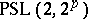,any prime;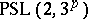,any odd number;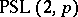,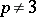a prime satisfying;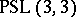; or

the Suzuki group,any odd prime. In particular, every finite minimal simple group is generated by two elements.

How to Cite This Entry:
Minimal simple group. Encyclopedia of Mathematics. URL: http://encyclopediaofmath.org/index.php?title=Minimal_simple_group&oldid=13947
This article was adapted from an original article by S.P. Strunkov (originator), which appeared in Encyclopedia of Mathematics - ISBN 1402006098. See original article